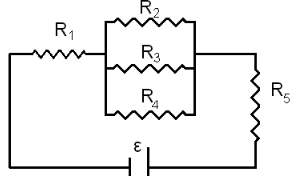# Problem: A circuit consisting of 5 resistors is shown in the graph. Their resistances are R1 = 17 Ω, R2 = 35 Ω, R3 = 86 Ω, R4 = 32 Ω, and R5 = 29 Ω, and the emf of the battery is ε = 5.5 V. Suppose the internal resistance of the battery is zero. Part (a) Express the equivalent resistance of the combination of R2, R3, and R4.Part (b) Express the total resistance of the circuit R in terms of R1, R2, R3, R4, and R5. Part (c) Calculate the numerical value of the total resistance R in ΩPart (d) Express the current I through R1 in terms of the emf ε and the equivalent resistance R. Part (e) Calculate the numerical value of I in A.Part (f) Express the power P dissipated by R1 through I and R1. Part (g) Calculate the numerical value of the power P in W.

###### FREE Expert Solution

Part (a)

Equivalent resistance for resistors in parallel:

$\overline{)\frac{\mathbf{1}}{{\mathbf{R}}_{\mathbf{eq}}}{\mathbf{=}}\frac{\mathbf{1}}{{\mathbf{R}}_{\mathbf{1}}}{\mathbf{+}}\frac{\mathbf{1}}{{\mathbf{R}}_{\mathbf{2}}}{\mathbf{+}}{\mathbf{.}}{\mathbf{.}}{\mathbf{.}}{\mathbf{+}}\frac{\mathbf{1}}{{\mathbf{R}}_{\mathbf{n}}}}$

1/R234 = 1/R2 + 1/R3 + 1/R4 = (R3R4 + R2R4 + R2R3)/R2R3R4

85% (259 ratings)###### Problem Details

A circuit consisting of 5 resistors is shown in the graph. Their resistances are R1 = 17 Ω, R2 = 35 Ω, R3 = 86 Ω, R4 = 32 Ω, and R5 = 29 Ω, and the emf of the battery is ε = 5.5 V. Suppose the internal resistance of the battery is zero.Part (a) Express the equivalent resistance of the combination of R2, R3, and R4.

Part (b) Express the total resistance of the circuit R in terms of R1, R2, R3, R4, and R5.

Part (c) Calculate the numerical value of the total resistance R in Ω

Part (d) Express the current I through R1 in terms of the emf ε and the equivalent resistance R.

Part (e) Calculate the numerical value of I in A.

Part (f) Express the power P dissipated by R1 through I and R1

Part (g) Calculate the numerical value of the power P in W.